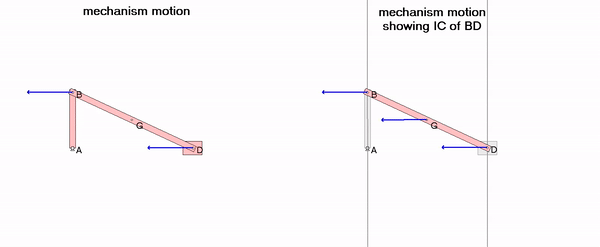# Instant centers: slider-crank mechanism

Consider the mechanism shown below made up of moveable links AB, BD and slider D. Link AB is rotating CCW at a constant rate. Point C is the center of rotation for BD, where point C is at the intersection of perpendiculars to the velocities of points B and D. Therefore, the velocity of any point on BD is perpendicular to the line connecting that point with C, and the speed of that point is proportional to the distance of that point from C. Since the location of the rotation center C moves with the mechanism motion, it is known as the “instant center” of rotation for BD. You can observe this in the video shown below.Watch the velocity of point G on link BD. The direction of this velocity is perpendicular to the line connecting G and C. This is true because at that instant, BD is rotating about the instant center C.

The direction of rotation of link BD changes between counterclockwise and clockwise at the two positions when link AB and DE are parallel (positions for which the instant center C is at ∞). Can you see this in the animation?